• 该软件为数学中的标准差公式，可以通过添加数组计算出平均值以及标准差
• 从两组的 n、均值和标准差计算合并的 n、均值和标准差（要计算为N个组（N> 2），请重复N-1次） [npool,meanpool,stdpool] = pooledmeanstd(n1,mean1,std1,n2,mean2,std2) 基于...matlab
• 所以这个就是对这个概念需要理解的一个点： 这里计算的是样本的标准偏差，总体标准偏差公式是基于正态分布推导而来，所以总体标准差公式是除以N，而在应用中，不是数学统计的意义，只能以有限的样本序列去近似描述...

[导读] 遇到一些朋友说信号处理真难，学是很辛苦的学了，就是不知道怎么用。学而不能致用，如此辛苦的学习就有点费时费力了。当然本文也并非想说学必致用，有的东西学了还真不见得能用上。只不过学过的，想用的要会用则达到学的目的了。此言：学以致用，学能致用！谨与诸君共勉！
很多时候，为什么学而不能致用呢？没有用的需求，当然就不说了。往往不会用，是因为不知道怎么去用，而不知道怎么用，个人觉得很重要的原因是因为很多基础的概念没有理解到位，对于工程技术人员而言，对于基础概念的理解把握，往往决定了解决问题的方向、思路、深度。以信号处理来说，里面就有大量的基础概念需要真正去理解。本文就来聊聊如何去描述度量信号的几个概念。
均值
信号处理中一个最为简单的概念就是均值(Mean)，和你想的一样，加起来除以样本数量：

在学习DSP时，要习惯各种数学表示的方案，比如这里

就是表示求和，

表示从

开始求和。为了让都能看懂，这个公式换一个表达形式：

所以

就是更为简洁的描述求和的数学语言。
对于这个公式在延申一下，这里是离散信号，如果是离散概率序列

,对于确定的

其概率为

，则这样的离散概率分布序列，其均值则为：

其实，对于前一公式也可以用概率均值去理解，看成N个样本集合，则每一个样值其概率就是

！
那么研究均值有啥意义呢？其实一般对于原始样本直接计算均值可能意义不是特别大，但是基于均值衍生的其他统计量则非常有价值，比如接下来要说的标准偏差，简称为标准差。
平均偏差
在谈标准差之前，先谈谈平均偏差。何为平均偏差，严格讲应该称为平均绝对偏差(Average Absolute Deviation)，在谈平均绝对偏差前，先谈谈绝对偏差,绝对偏差，从字面意义上理解，很容易可以想到其计算这样是这样得来，由某样本与均值的差的绝对值：

那么平均绝对偏差，所差的就是一个平均了：

来试着理解一下这个公式，

是任一样本

与该样本集均值的差的绝对值，表示的是该样本

与均值的偏离程度，每个样本与均值的偏离程度之和再求平均，则就是字面意思了，所有样本与平均值的偏离程度，故称为平均偏差。

平均偏差可以反应样本点与均值的平均偏离程度。
标准偏差
标准偏差(Standard Deviation)与平均偏差(Average Deviation)类似，也是基于平均值的统计量。所不同的是，标准差是利用样本与均值绝对偏差的平方和求取的。
标准差反应信号相对平均值的波动程度。标准差数值越小，反应信号数值分布更靠近平均值，反之越大则表示信号相对平均值更分散
标准偏差根据样本是研究样本的总体，还是只是收集的部分样本而分为两种情况：
总体标准偏差样本标准偏差
总体标准偏差
如果仅将数据视为总体，则可以将其各点绝对偏差之和除以数据点总数N，而后开平方：

样本标准偏差
如果待研究的数据看成待研究系统数据的部分，则可以将其各点绝对偏差之和除以数据点总数N-1，而后开平方：

看到这个公式，有的盆友或许会问，为啥除的是N-1？而不是N！所以这个就是对这个概念需要理解的一个点：

这里计算的是样本的标准偏差，总体标准偏差公式是基于正态分布推导而来，所以总体标准差公式是除以N，而在应用中，不是数学统计的意义，只能以有限的样本序列去近似描述总体的特征，除以N-1是一种无偏估计，所谓无偏估计，是指无偏性，无偏性的实际意义是指没有系统性的偏差。在多次重复下，它们的平均数接近所估计的参数真值。

我们计算这个参数，就是想利用这个参数去反应样本序列集的客观特征，所计算的样本序列往往可能只是截取的数据段，并非所有的数据样本。在信号处理中，我们拿到的数据一般而言都是系统的部分样本，所以实际使用中应该使用样本标准差进行计算。
对于标准偏差的理解，还有一层需要理解透，它的量纲仍然是原样本的量纲，比如研究的是电压信号，单位为伏，则计算而得的标准偏差依然是伏。
有趣的栗子
在国外网站上看到一组有趣的图片，可以更好的帮助理解：
https://www.mathsisfun.com/data/standard-deviation.html
假设有这样几种可爱的狗狗：其身高分别为：600mm, 470mm, 170mm, 430mm, 300mm.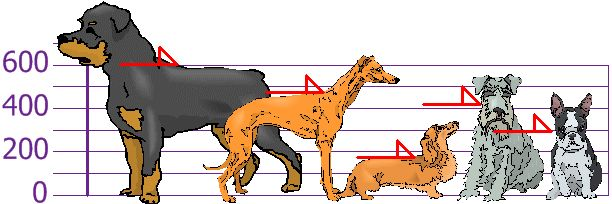则其均值为：

所以上图中用绿色线标识下身高均值：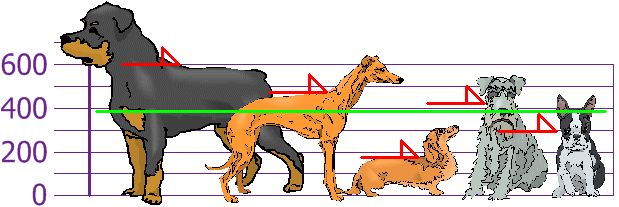从而每个狗相对均值的偏差如下图：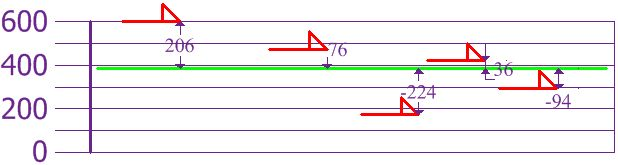从而，其标准差则为：

然后再标识一下每个狗的身高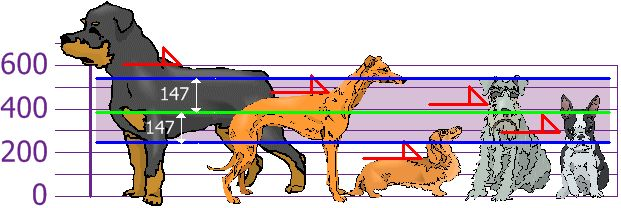上图可看出第2、4、5个狗的身高与均值的偏差在一个标准差内，而第1、3只狗身高与均值超出了一个标准差。标准差概念也经常用来衡量产品的生成品质，比如你常听到的说法，这个零件的加工偏差是否在一个标准差内，这里的标准差就是标准偏差的意思。
上面的公式如果不开平方，这就是常说的方差了，类似有两种概念：
样本方差：

总体方差：

再来个栗子
前面说标准差，常用来衡量数据的分布情况：

标准差反应信号相对平均值的波动程度。标准差数值越小，反应信号数值分布更靠近平均值，反之越大则表示信号相对平均值更分散

为啥这样说，看看下面这个栗子就好理解了：
假设有这样三组数据，假定这三组数据来自三个同类型传感器的采样值，对相同的外界多次采样(这里为了说明问题，请不用考虑数据本身的合理性)，我们来计算一下其均值、平均偏差、样本标准差。

1357911131517192457891315132435577810121330

三组数据连同其均值绘制成曲线：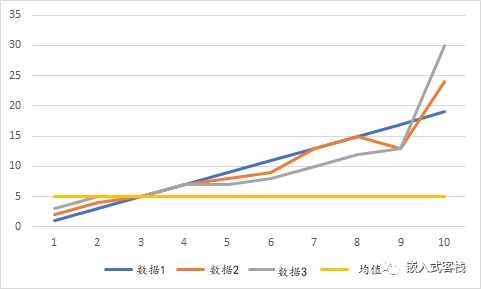第1组：

第2组：

第3组：

从曲线图我们可以很直观的看出第1个传感器表现更好，那么如何用一个特征值来区分呢？如用平均绝对偏差显然并不能很好的描述，三组数据均值相同，无法区分三个传感器的表现，因为计算出平均绝对偏差相同。如用样本标准差进行度量，则可以得出：

其物理含义，表示第1组数据分布程度相对更为靠近平均值。
总结一下
均值、平均偏差、标准偏差、方差是信号处理几个基础概念，尤其标准差、方差在很多复杂的滤波算法、估计算法中是重要的理论基础概念。所以准确的理解这些概念，也是能理解更为复杂的算法的基础。所谓基础不牢、地动山摇！
—END—
往期精彩推荐，点击即可阅读
▲Linux驱动相关专辑
▲手把手教信号处理专辑
▲单片机相关专辑

展开全文• 平均分布下的标准差公式计算方法，统计学的基础知识，包含密度函数，分布函数推导过程，是人工智能的数据分析的基础知识统计学
• 很精巧的平均数、方差、标准差计算器。免去自己计算的麻烦，手工输入数据，然后一键计算！
• 稳健性是估计算法的特点，而不是其产生的估计值的特点，因此严格来说，称由此算法计算的平均和标准差是稳健的是不确切的。然而，为避免使用繁琐的术语，“稳健均值”“稳健标准差”应理解为利用稳健算法计算的...


文章目录
算法原理代码

本文主要参考 ISO 13528 的附件 C 的 3 条款。

算法原理
应用此算法计算得到数据平均值和标准差的稳健值。稳健性是估计算法的特点，而不是其产生的估计值的特点，因此严格来说，称由此算法计算的平均值和标准差是稳健的是不确切的。然而，为避免使用繁琐的术语，“稳健均值”和“稳健标准差”应理解为利用稳健算法计算的总体均值和总体标准差的均值估计。
设数据为

x

i

,

i

∈

(

1

,

2

,

⋯

,

n

)

x_i, i\in(1,2,\cdots,n)

，记稳健平均值为

x

∗

,

s

∗

x^*, s^*

，首先是计算初始值：

x

∗

=

med

⁡

x

i

s

∗

=

1.483

×

med

⁡

∣

x

i

−

x

∗

∣

\begin{array}{c} x^{*}=\operatorname{med} x_{i} \\ s^{*}=1.483 \times \operatorname{med}\left|x_{i}-x^{*}\right| \end{array}

对每个

x

i

x_i

，有：

x

i

∗

=

{

x

∗

−

δ

,

若

x

i

<

x

∗

−

δ

x

∗

+

δ

,

若

x

i

>

x

∗

+

δ

x

i

,

其他

x_{i}^{*}=\left\{\begin{array}{cc} x^{*}-\delta, & \text { 若 } x_{i}<x^{*}-\delta \\ x^{*}+\delta, & \text { 若 } x_{i}>x^{*}+\delta \\ x_{i}, & \text { 其他 } \end{array}\right.

其中：

δ

=

1.5

s

∗

\delta=1.5 s^{*}

，再次计算：

x

∗

=

∑

x

i

∗

/

p

s

∗

=

1.134

∑

(

x

i

∗

−

x

∗

)

2

/

(

p

−

1

)

\begin{array}{c} x^{*}=\sum x_{\mathrm{i}}^{*} / p \\ s^{*}=1.134 \sqrt{\sum\left(x_{\mathrm{i}}^{*}-x^{*}\right)^{2} /(p-1)} \end{array}

重复：

x

i

∗

=

{

x

∗

−

δ

,

若

x

i

<

x

∗

−

δ

x

∗

+

δ

,

若

x

i

>

x

∗

+

δ

x

i

,

其他

x_{i}^{*}=\left\{\begin{array}{cc} x^{*}-\delta, & \text { 若 } x_{i}<x^{*}-\delta \\ x^{*}+\delta, & \text { 若 } x_{i}>x^{*}+\delta \\ x_{i}, & \text { 其他 } \end{array}\right.

直到

s

∗

s^*

的 第三位有效数字和

x

∗

x^*

的对应数字在连续两次迭代中不变。
代码
# -*- coding: utf-8 -*-
import pandas as pd
import numpy as np

def location_corresponding(x_str, s_str):
'''
s 的第三位有效数字，以及对应 x 的相应的数字
'''
digit_location = s_str.find('.')
if digit_location >= 3:
# 如果小数点在字符串的第 4 位或大于第四位，也即数字至少在 100 以上
# 此时第三位有效数字，肯定在小数点前。
significant_num = s_str
# 有效位数于小数点的相对位置
# 0 代表在小数点前， 3-1 是指从小数点前数起的位数
# sig_loc 的第一位表示有效数字在小数点前，还是后
# 第二位代表有效数字在小数点的“距离”
str_len = len(s_str[:digit_location])
sig_loc = (0, str_len-3-1)

elif s_str == '0':
# 若整数部分是0，则有效数字的位置在小数点后
# 刨除整数和小数点部分
s_without_int = s_str[digit_location:]
# 若小数点后有 0，则不将 0 计入有效数字位
if '.0' in s_without_int:
digit_location += 1
while '.00' in s_without_int:
# 若小数点后有多个0，也不计入
s_without_int = s_without_int.replace('.00', '.0')
digit_location += 1
try:
# 若位数不够，如 0.0，则有效数字为 0、
significant_num = s_str[digit_location+3]
except:
significant_num = '0'
# 1 表示有效数字在小数点后
sig_loc = (1, 3)

elif digit_location == -1 and len(s_str) >= 3:
# 若只有整数，没有小数部分，且整数部分大于 100，即有超过三位数
# 则直接取第三位
str_len = len(s_str)
sig_loc = (0, str_len-3)
significant_num = s_str
elif digit_location == -1 and len(s_str) < 3:
# 若小于 100，则有效数字为0
significant_num = '0'
else:
# 若整数部分小于 2 位，且整数部分大于 0
sig_loc = (1, 3-digit_location)
significant_num = s_str[sig_loc+digit_location]

# x 对应的数字
x_digit_location = x_str.find('.')
if sig_loc == 0:
if x_digit_location == -1 and len(x_str) >= 3:
x_significant_num = x_str
elif x_digit_location == -1 and len(x_str) < 3:
x_significant_num = '0'
else:
x_significant_num = x_str[x_digit_location-sig_loc]
print(sig_loc)
print('前')
else:
try:
x_significant_num = x_str[x_digit_location+sig_loc]
except:
x_significant_num = '0'

return x_significant_num, significant_num

def coverage_critiria(x_list, s_list):
'''
收敛准则
其中 s_list 是一个长度为 3 的 list, 包含当前迭代的 s* 和之前两个迭代的 s*
其中 x_list 也一样

难点在于：如何找出 s 的第三位有效数字，对应 x 的数位呢？
这里的解决办法是：找出 s 三位有效数字，在小数点的位置，从而应用于 x 中
'''

s_numbers = []
x_numbers = []
for i in range(3):
# 连续两位不变，故需要进行 3 此迭代。
s = s_list[i]
# s 的字符串
s_str = str(s)

x = x_list[i]
# x 的字符串
x_str = str(x)
# 找出 s* 的第三位有效数字，和 x* 对应的数字。
x_sig_num, s_sig_num = location_corresponding(x_str, s_str)
s_numbers.append(s_sig_num)
x_numbers.append(x_sig_num)

# 稳健标准差的第三位有效数字，连续两次不变，且稳健平均值的对应数字亦连续两次不变，则判断为收敛
# 函数返回 True。否则返回 False
s_equal_flag = (s_numbers == s_numbers) and (s_numbers == s_numbers)
x_equal_flag = (x_numbers == x_numbers) \
and (x_numbers == x_numbers)

if s_equal_flag and x_equal_flag:
return True
else:
return False

def perform_algorithm_A(x):
'''
x 是一个向量
'''
if type(x) is list:
x = np.array(x)

# 稳健平均和稳健标准差初始值
x_star = np.median(x)
x_diff = np.absolute(x-x_star)
s_star = 1.483*np.median(x_diff)

# 上界和下界的初始值
delta = 1.5*s_star
higher_bound = x_star + delta
lower_bound =  x_star - delta

x_list = []
s_list = []
while True:
x_tmp = np.copy(x)
x_tmp[x_tmp>higher_bound] = higher_bound
x_tmp[x_tmp<lower_bound] = lower_bound
x_star = np.mean(x_tmp)
s_star = 1.134*np.std(x_tmp)

x_list.append(x_star)
s_list.append(s_star)

if len(x_list) == 4:
x_list.pop(0)
s_list.pop(0)
# 达到收敛条件
if coverage_critiria(x_list, s_list):
return x_tmp, x_list, s_list, higher_bound, lower_bound

# 计算上下界
delta = 1.5*s_star
higher_bound = x_star + delta
lower_bound =  x_star - delta

if __name__ == '__main__':
x = [927,952,977,995,915,962,966,950,969,949,961,940,1002,956,960,943]
x, x_star, s_star, higher_bound, lower_bound = perform_algorithm_A(x)
print('算法 A 收敛后，数据变为： ', x)
print('稳健平均值： ', x_star)
print('稳健标准差： ', s_star)


运行结果如下：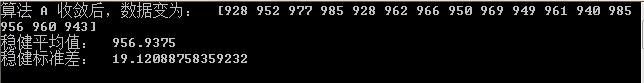展开全文算法A
• Cpk:制程能力指标 Cp: 技术能力指标 k: 管理能力指标 Mean:平均值 s: 标准差,值越大，数据越散乱 USL:规格上限 LSL:规格下限
• 今天小编就为大家分享一篇Python求均值,方差,标准差的实例，具有很好的参考价值，希望对大家有所帮助。一起跟随小编过来看看吧
• 标准误概念：即样本均数的标准差，可用于衡量抽样误差的大小。 通常σ未知，用s（标准差）来估计，计算标准误： ----> 通过增加样本含量n来降低抽样误差。 标准误的特点： 当样本例数n一定时，标准误与...
标准误概念：即样本均数的标准差，可用于衡量抽样误差的大小。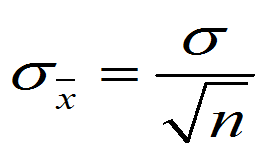通常σ未知，用s（标准差）来估计，计算标准误：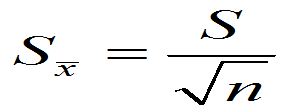---->    通过增加样本含量n来降低抽样误差。

标准误的特点：
当样本例数n一定时，标准误与标准差呈正比；
当标准差一定时，标准误与样本含量n的平方根呈反比。
意义：反映样本均数间离散程度。反映抽样误差的大小。标准误越小，抽样误差越小，用样本均数估计总体均数的可靠性越大。

标准差和标准误的差别：
标准误：反映抽样误差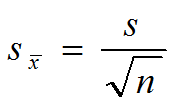用于总体均数、置信区间、进行统计学检验
标准差：反映个体变异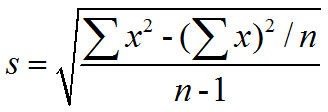用于计算参考值范围、计算标准误、CV
联系：当n一定时，标准差越大，标准误越大
展开全文统计分析 标准误
• 移动标准差以及移动平均值(movstd、movmean) 最近在工作中遇到这样一个问题： 有一个序列长度为 nnn 的序列 T=[t0,t1,…,tn−1]T=[t_0, t_1, \dots, t_{n-1}]T=[t0​,t1​,…,tn−1​]，给定一个窗大小 m(m<=n)m ...
移动标准差以及移动平均值(movstd、movmean)
最近在工作中遇到这样一个问题： 有一个序列长度为

n

n

的序列

T

=

[

t

0

,

t

1

,

…

,

t

n

−

1

]

T=[t_0, t_1, \dots, t_{n-1}]

，给定一个窗大小

m

(

m

<

=

n

)

m (m <= n)

，下标从0开始，计算窗大小的均值和标准差，即计算T[0:m-1]、T[1:m]、T[2:m+1]…T[n-m+1:n] 的平均值和标准差暴力解法
最简单的无脑的方法就是暴力循环了，很明显这种方法特别慢，时间复杂度为

O

(

n

∗

m

)

O(n*m)

下面为你呈现暴力代码
import numpy as np
import time

# generate time sequence
n = 1000 * 1000
m = 1000
T = np.random.rand(n)

# brute force
means = np.zeros(n - m + 1)
stds = np.zeros(n - m + 1)

start_time = time.time()
for i in range(n - m + 1):
means[i] = np.mean( T[i:i+m] )
end_time = time.time()
print('Running time of brute force for mean is {}s'.format((end_time - start_time)))

start_time = time.time()
for i in range(n - m + 1):
stds[i] = np.std( T[i:i+m] )
end_time = time.time()
print('Running time of brute force for std is {}s'.format((end_time - start_time)))

Running time of brute force for mean is 5.774143934249878s
Running time of brute force for std is 20.721827030181885s

movmean
有没有办法进行优化呢？这里介绍移动标准差(movstd)和移动平均值(movmean)
先从移动平均值(movmean)开始，它很简单并且符合直觉：在滑动的过程中，有很多重叠部分，我们可以利用重叠的部分，从而节约计算时间如上图所示，计算时可以利用前一个均值，这样就避免了不必要的加法操作，平均值的计算复杂度降低为

O

(

n

)

O(n)

μ

i

∗

m

=

(

t

i

+

t

i

+

1

+

⋯

+

t

i

+

m

−

1

)

\mu_i*m = (t_i + t_{i+1} + \dots + t_{i+m-1})

μ

i

+

1

∗

m

=

μ

i

∗

m

−

t

i

+

t

m

\mu_{i+1}*m = \mu_i*m - t_i + t_m

下面代码显示了如何实现 movmean
def movmean(T, m):
assert(m <= T.shape)
n = T.shape

sums = np.zeros(n - m + 1)
sums = np.sum(T[0:m])

cumsum = np.cumsum(T)
cumsum = np.insert(cumsum, 0, 0) # 在数组开头插入一个0

sums = cumsum[m:] - cumsum[:-m]
return sums/m

start_time = time.time()
means_2 = movmean(T, m)
end_time = time.time()
print('Running time of movmean is {}s'.format((end_time - start_time)))

Running time of movmean is 0.009449005126953125s

movstd
在介绍移动标准差之前，我们先回顾下标准差计算公式：

σ

=

1

m

∑

i

=

1

m

(

t

i

−

u

)

2

\sigma = \sqrt{\frac{1}{m} \sum_{i=1}^m(t_i - u)^2}

假设有一个长度为 3 的序列 [a, b, c]，我们来计算一下它的标准差
首先计算均值：

μ

=

1

m

(

a

+

b

+

c

)

\mu = \frac{1}{m}(a+b+c)

然后标准差：

σ

=

1

3

(

(

a

−

μ

)

2

+

(

b

−

μ

)

2

+

(

c

−

μ

)

2

)

=

1

3

(

a

2

+

b

2

+

c

2

−

2

a

μ

−

2

b

μ

−

2

c

μ

+

μ

2

)

=

1

3

(

a

2

+

b

2

+

c

2

)

−

(

1

3

(

a

+

b

+

c

)

)

2

\begin{array}{l} \sigma &= \sqrt{ \frac{1}{3} ((a-\mu)^2 + (b-\mu)^2 + (c-\mu)^2)} \\ &= \sqrt{ \frac{1}{3} (a^2 + b^2 + c^2 -2a\mu - 2b\mu - 2c\mu + \mu^2)} \\ &= \sqrt{ \frac{1}{3}(a^2+b^2+c^2) - (\frac{1}{3}(a+b+c))^2 } \\ \end{array}

我们可以发现，标准差的计算可以用累计和来表示，而累加和是可以在

O

(

n

)

O(n)

时间内完成，这就是 movstd
下面的代码展示了如何计算 movstd
def movstd(T, m):
n = T.shape

cumsum = np.cumsum(T)
cumsum_square = np.cumsum(T**2)

cumsum = np.insert(cumsum, 0, 0)               # 在数组开头插入一个0
cumsum_square = np.insert(cumsum_square, 0, 0) # 在数组开头插入一个0

seg_sum = cumsum[m:] - cumsum[:-m]
seg_sum_square = cumsum_square[m:] - cumsum_square[:-m]

return np.sqrt( seg_sum_square/m - (seg_sum/m)**2 )

start_time = time.time()
stds_2 = movstd(T, m)
end_time = time.time()
print('Running time of movstd is {}s'.format((end_time - start_time)))

Running time of movstd is 0.03198814392089844s

总结
通过提前计算好累计和，移动平均和移动标准差以空间换时间，算法速度比起暴力方法提升了几个数量级
展开全文• matlab标准差公式代码目录 挑战总结 想象您在一家电子商务公司Market-ter上，该公司也有一个社交网络。 除了购物外，用户还可以查看其朋友正在购买哪些商品，并受其网络中的购买影响。 您面临的挑战是建立一个实时...
• 假设有一组序列，期望以n为步进，滑动求得每n个数据的平均和标准差（即，想要求得，的平均和标准差，的平均和标准差，以此类推）。那么，我们期望通过一种递推公式，来减少计算量。 先上结论（注：用方差替代...统计学 数学
• 如图，论文中，一般都会给出平均和标准差。 大家在写科技论文的时候，通常用excel来整理数据，那如何用excel快速实现“平均值±标准差”。 要Tina说，这很简单！ 记住下面这个公式即可。 ROUND(AVERAGE(B2:Bx),4...
• 直接上代码 #调出numpy import numpy as np arr = [2, 4, 6, 8, 10, 12] # 要计算的数值 # 求均值 arr_mean = np.mean(arr) ...arr_var = np.var(arr) # 求标准差 ...print("标准差为:%f" % arr_stpython
• ## 标准差公式的变形

千次阅读 2016-04-07 20:19:00
标准差的一般求法是：但是这样在程序中每次xi都减去x，不方便。 根据以上公式可以推导出： 标准差的平方=segem（xi*xi）/n-x*x；（x是平均值）。 推导过程如下： 将标准形式的segem（）展开后得到：1.segem（xi...
• 百度+猜测认为误差线是平均值±标准差 用PDF快速看图量了一下，官网链接点这里 excel公式为 =NORMINV(RAND(),$D2,$E2) 其中D2是均值，E2是标准差，接下来只要复制就会有一系列数据了。 最后复制粘贴到原位置只保留...
• 计算列表中所有数的平均和标准差。 代码 import random def mean(lst): s = sum(lst) n = len(lst) return s/n def sd(lst): import math mean = sum(lst)/len(lst) bias_mean = [(x - mean)**2 for x in ...python
• 总之，最后我们得到了修正的标准差的（预计）公式（称为样本标准差）： 标准差(Standard Deviation) 标准误差(Standard Error) 顺带一下，不要直接使用此公式计算SD，会产生非常多舍入误差(rounding error)...标准误
• 上面的式子中，我们需要准确的了解随机变量X的总体分布，从而可以计算出其总体的期望和标准差。 但在一般情况下，对总体的每一个个体都进行观察或试验是不可能的。因此，必须对总体进行抽样观察（采样）。由于我们...统计学
• 标准差和方差 测量狗的高度(毫米) 高度（在肩部）为：600mm，470mm，170mm，430mm300mm。 找出均值（Mean），方差（Variance）标准偏差(（Standard Deviation）。 mean在就是average的意思表示平均水平 第一步...variance
• catalog平均数算术平均数几何平均数调和平均数算术-几何平均标准差 平均数 比如我们有n个数据： {1, 2, 3, 4, ...} 我们想要得到 “一个常数C”，用这1个常数C 就可以代表这个n个数据！ 我们以往只知道 用： C =...
• 计算C语言中的求和、标准差、方差和标准差等，需要加上头文件：#include <math.h> #include<stdio.h> #include "math.h" double sum = 0;//求和 double array = {1.2,2.1,3.1,4.1}; int length...
• ## 平均数和标准差

千次阅读 2013-10-11 19:58:46
• 单纯介绍概念不易理解，所以应从实际应用出发介绍其区别。 四者的研究对象研究目的不同。...定义：标准差是观测值与其平均偏差的平方的平方根，即方差的算术平方根。 公式: 公式意义：所有数减去其...均方差 区别
• 一、离差(Deviation) 离差即标志变动度，又称“偏差”，是观测值或估计量的平均值与真实值之间的差，是反映数据分布离散程度的量度之一，或说是反映统计总体中各单位标志值差别大小...平均差也称为均值，是数据分布中所人工智能 数据分析
• 标准差SD、相对标准偏差RSD学习python实现前言一、SDRSD的定义、公式、深层意义（1）定义（2）公式（3）RSD的必要性二、Python实现1.SD2.读入数据总结 前言 提示：这里可以添加本文要记录的大概内容： 例如：...数据分析 python
• // 定义两个简单的函数 let sum = (x,y) =>...// 然后将这些函数数组方法配合使用 计算出平均和标准差 let data = [1, 1, 3, 5, 5] let mean = data.reduce(sum)/data.length; let deviations =...
• 相对标准平方偏差计算软件，祝你RSD=99.99%，亲测好用
• 一、定义、公式二、方差、标准差 vs 协方差、相关系数 区别一、定义、...2、标准差定义：标准差（Standard Deviation） ，是离均差平方的算术平均数的算术平方根，用σ表示。标准差也被称为标准偏差，或者实验标准差......